• 归并排序的时间复杂度推到
千次阅读
2021-05-24 21:53:41

归并排序算法你还记得吧？它的递归实现代码非常简洁。现在我们就借助归并排序来看看，如何用递归树，来分析递归代码的时间复杂度。

归并排序每次会将数据规模一分为二。我们把归并排序画成递归树，就是下面这个样子：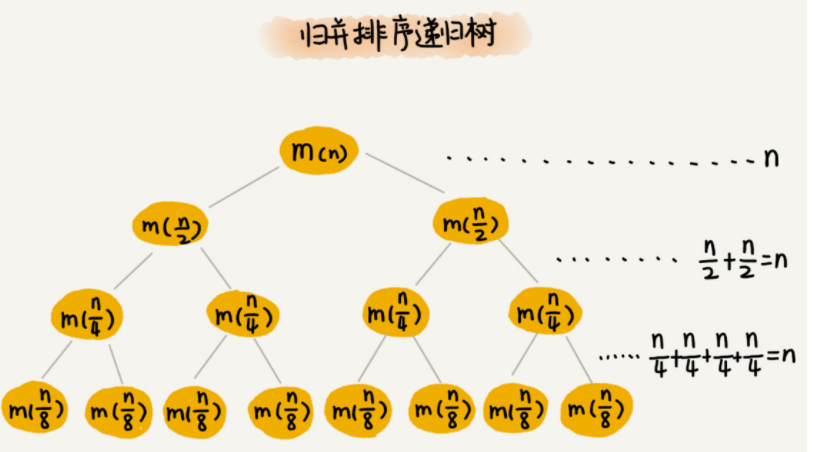因为每次分解都是一分为二，所以代价很低，我们把时间上的消耗记作常量 1。归并算法中比较耗时的是归并操作，也就是把两个子数组合并为大数组。从图中我们可以看出，每一层归并操作消耗的时间总和是一样的，跟要排序的数据规模有关。我们把每一层归并操作消耗的时间记作 n。

现在，我们只需要知道这棵树的高度 h，用高度 h 乘以每一层的时间消耗 n，就可以得到总的时间复杂度 O(n∗h)。

从归并排序的原理和递归树，可以看出来，归并排序递归树是一棵满二叉树。我们前两节中讲到，满二叉树的高度大约是 log2​n，所以，归并排序递归实现的时间复杂度就是 O(nlogn)。我这里的时间复杂度都是估算的，对树的高度的计算也没有那么精确，但是这并不影响复杂度的计算结果。

## 总结

• 归并排序的时间复杂读为nlogn,每一行时间复杂度O(n) 然后二叉树高度log(n)

## 参考

归并排序图解_鸭梨的博客-CSDN博客归并排序 递归树
更多相关内容
• 根号n段归并排序算法时间复杂度分析过程： 1.合并 根号n向下取整 段子数组使用的是自底向上两两归并的策略 2.根号n段归并排序算法时间复杂度的数学推导
• ## 归并排序时间复杂度分析

万次阅读 多人点赞 2019-03-19 18:04:57
归并排序时间复杂度分析归并排序工作原理时间复杂度计算如何插入一段漂亮的代码片KaTeX数学公式 归并排序 归并排序也叫（Merge sort）。 工作原理 将给定的数组一份为二 对两部分数组再使用归并排序使其有序 最后再...

# 归并排序

归并排序也叫（Merge sort）。

## 工作原理

1. 将给定的数组一份为二
2. 对两部分数组再使用归并排序使其有序
3. 最后再将两部分数组合并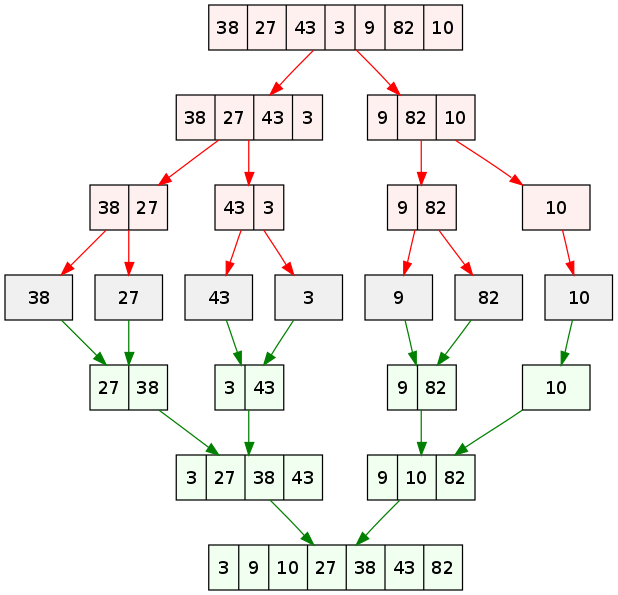## 时间复杂度计算

1、首先可知

f ( n ) = 2 f ( n 2 ) + n           f(n)=2f(\frac{n}{2})+n \space\space\space\space\space\space\space\space\space
其中： f ( n ) 表 示 对 n 个 数 进 行 归 并 排 序 f(n)表示对n个数进行归并排序 2 f ( n 2 ) 表 示 将 n 个 数 分 成 两 部 分 分 别 进 行 归 并 排 序 2f(\frac{n}{2})表示将n个数分成两部分分别进行归并排序 n 表 示 对 两 个 子 过 程 结 束 之 后 合 并 的 过 程 n表示对两个子过程结束之后合并的过程
2、推导
f ( n 2 ) = 2 f ( n 4 ) + n 2           当 n = n 2 时 f(\frac{n}{2})=2f(\frac{n}{4})+\frac{n}{2} \space\space\space\space\space\space\space\space\space 当n=\frac{n}{2}时
f ( n 4 ) = 2 f ( n 8 ) + n 4           当 n = n 4 时 f(\frac{n}{4})=2f(\frac{n}{8})+\frac{n}{4} \space\space\space\space\space\space\space\space\space 当n=\frac{n}{4}时
… …           …… \space\space\space\space\space\space\space\space\space
f ( n 2 m − 1 ) = 2 f ( n 2 m ) + n 2 m − 1           当 n = n 2 m − 1 时 f(\frac{n}{2^{m-1}})=2f(\frac{n}{2^m})+\frac{n}{2^{m-1}} \space\space\space\space\space\space\space\space\space 当n=\frac{n}{2^{m-1}}时
3、由此可得：

f ( n ) = 2 f ( n 2 ) + n f(n)=2f(\frac{n}{2})+n
= 2 × ( 2 f ( n 4 ) + n 2 ) + n = 2\times\bigg(2f(\frac{n}{4})+\frac{n}{2}\bigg)+n
= 2 2 f ( n 2 2 ) + 2 n =2^2f(\frac{n}{2^2})+2n
= 2 2 × ( 2 f ( n 8 ) + n 4 ) + 2 n =2^2\times\bigg(2f(\frac{n}{8})+\frac{n}{4}\bigg)+2n
= 2 3 f ( n 2 3 ) + 3 n =2^3f(\frac{n}{2^3})+3n
… … ……
= 2 m f ( n 2 m ) + m n =2^mf(\frac{n}{2^m})+mn

当 m 足够大时(仅剩一个数字时)，可使得 n 2 m = 1 \frac{n}{2^m}=1
求出 m = l o g 2 n m=log_2n

代 入    f ( n ) = 2 m f ( n 2 m ) + m n    中 可 得 代入 \space\space f(n)=2^mf(\frac{n}{2^m})+mn \space\space 中可得
f ( n ) = 2 ( l o g 2 n ) f ( 1 ) + n ⋅ l o g 2 n f(n)=2^{(log_2n)}f(1)+n\cdot log_2n
其 中    f ( 1 ) = 0 其中\space\space f(1)=0
所 以 最 终    f ( n ) = n ⋅ l o g 2 n 所以最终\space\space f(n)=n\cdot log_2n

展开全文排序算法 分治算法
• ## 归并排序时间复杂度过程分析

万次阅读 多人点赞 2019-07-08 20:54:43
归并排序方法就是把一组n个数的序列，折半分为两个序列，然后再将这两个序列再分，一直分下去，直到分为n个长度为1的...归并排序时间=分解时间+子序列排好序时间+合并时间 无论每个序列有多少数都是折中分解，...

https://blog.csdn.net/liangjiabao5555/article/details/89670082

归并排序方法就是把一组n个数的序列，折半分为两个序列，然后再将这两个序列再分，一直分下去，直到分为n个长度为1的序列。然后两两按大小归并。如此反复，直到最后形成包含n个数的一个数组。

归并排序总时间=分解时间+子序列排好序时间+合并时间

无论每个序列有多少数都是折中分解，所以分解时间是个常数，可以忽略不计。

则：归并排序总时间=子序列排好序时间+合并时间

如果假设一个序列有n个数的排序时间为T(n)，T(n)是一个关于n的函数，随着n的变化而变化。

那么我们将n个数的序列，分为两个(n/2)的序列。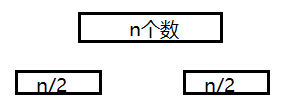那么T(n)=2*T(n/2)+合并时间

由于合并时，两个子序列已经组内排好序了，那我们将两个排好序的序列组合成一个大的有序序列，只需要一个if循环即可。if循环中有n个数需要比较，所以时间复杂度为n。

那么T(n)=2*T(n/2)+n

我们再将两个n/2个序列再分成4个(n/4)的序列。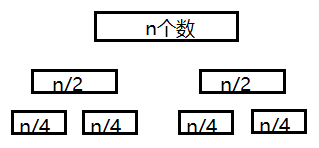一个（n/2）序列排序时间=两个(n/4)的序列排序时间+两个(n/4)的序列的合并为一个（n/2）的序列时间

T(n/2)=2*T(n/4)+n/2

将T(n/2)带入到T(n)中，T(n)=2*(2*T(n/4)+n/2)+n，

通过化简T(n)=4*T(n/4)+2n

我们再将4个n/4个序列再分成8个(n/8)的序列。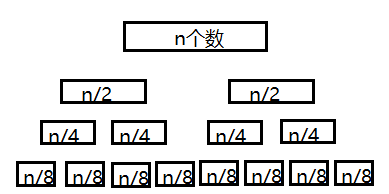T(n/4)=2*T(n/8)+n/4

将T(n/4)带入到黄色公式中，T(n)=4*(2*T(n/8)+n/4)+2n

通过化简T(n)=8*T(n/8)+3n

······

这样分下去，前面我们已经说过了，分为n个序列，每个序列里只有一个数为止。

前面我们假设的一个序列有n个数的排序时间为T(n)，现在每个序列只有一个数，所以不需要进行组内排序，时间复杂度为0。T(1)=0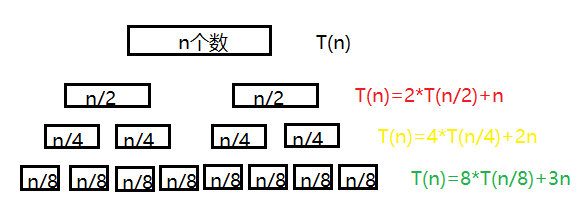大家有没有找到规律，右边式子中n前面的系数随着层数的增加而增加，第一层公式中没有n，则系数为0，第二层n的系数为1，第三层为2，第四层为3。那么规律就出来了，n前面的系数为层数减1。

那这个图有没有很熟悉，就像一个二叉树一样，通过二叉树的知识点我们可以知道，一个n个结点的二叉树层数为(log2n)+1。

那么n前面的系数为层数减1。

(log2n)+1-1=log2n

那log2n就是最底层n的系数。

那么我们最后一层是不是可以这样表示

T(n)=n*T(1)+(log2n)*n

T(1)=0，那么T(n)=(log2n)*n

所以归并排序的时间复杂度为nlog2n

展开全文• 归并排序时间=分解时间+子序列排好序时间+合并时间 无论每个序列有多少数都是折中分解，所以分解时间是个常数，可以忽略不计。 则：归并排序时间=子序列排好序时间+合并时间 如果假设一个序列有n个数的排序...

归并排序方法就是把一组n个数的序列，折半分为两个序列，然后再将这两个序列再分，一直分下去，直到分为n个长度为1的序列。然后两两按大小归并。如此反复，直到最后形成包含n个数的一个数组。

归并排序总时间=分解时间+子序列排好序时间+合并时间

无论每个序列有多少数都是折中分解，所以分解时间是个常数，可以忽略不计。

则：归并排序总时间=子序列排好序时间+合并时间

如果假设一个序列有n个数的排序时间为T(n)，T(n)是一个关于n的函数，随着n的变化而变化。

那么我们将n个数的序列，分为两个(n/2)的序列。那么T(n)=2*T(n/2)+合并时间

由于合并时，两个子序列已经组内排好序了，那我们将两个排好序的序列组合成一个大的有序序列，只需要一个if循环即可。if循环中有n个数需要比较，所以时间复杂度为n。

那么T(n)=2*T(n/2)+n

我们再将两个n/2个序列再分成4个(n/4)的序列。一个（n/2）序列排序时间=两个(n/4)的序列排序时间+两个(n/4)的序列的合并为一个（n/2）的序列时间

T(n/2)=2*T(n/4)+n/2

将T(n/2)带入到T(n)中，T(n)=2*(2*T(n/4)+n/2)+n，

通过化简T(n)=4*T(n/4)+2n

我们再将4个n/4个序列再分成8个(n/8)的序列。T(n/4)=2*T(n/8)+n/4

将T(n/4)带入到黄色公式中，T(n)=4*(2*T(n/8)+n/4)+2n

通过化简T(n)=8*T(n/8)+3n

······

这样分下去，前面我们已经说过了，分为n个序列，每个序列里只有一个数为止。

前面我们假设的一个序列有n个数的排序时间为T(n)，现在每个序列只有一个数，所以不需要进行组内排序，时间复杂度为0。T(1)=0大家有没有找到规律，右边式子中n前面的系数随着层数的增加而增加，第一层公式中没有n，则系数为0，第二层n的系数为1，第三层为2，第四层为3。那么规律就出来了，n前面的系数为层数减1。

那这个图有没有很熟悉，就像一个二叉树一样，通过二叉树的知识点我们可以知道，一个n个结点的完全二叉树层数为(log2n)+1。

那么n前面的系数为层数减1。

(log2n)+1-1=log2n

那log2n就是最底层n的系数。

那么我们最后一层是不是可以这样表示

T(n)=n*T(1)+(log2n)*n

T(1)=0，那么T(n)=(log2n)*n

所以归并排序的时间复杂度为nlog2n

展开全文数据结构 排序算法
• 归并排序算法 设置归并排序所化时间为 T(N)T(N)T(N)，其中 NNN 为输入数据长度 ...4、归并排序好的左边和右边元素，时间复杂度 θ(N)\theta(N)θ(N) 设置归并排序所化时间为 T(N)T(N)T(N)，其中 NNN 为输入
• 今天讲讲，为什么归并排序时间复杂度是θ(nlgn) 我们知道归并排序是利用 递归函数 进行计算的一种分治排序算法，因此对其时间复杂度也可以用递归的方式进行计算。 首先我们要知道分治算法的流程： 分解、计算（将...
•java 经典排序算法 归并排序 稳定性
•归并排序 排序
• 引言：（一）快速排序的最好情况O（nlogn...此时就和归并排序基本一致了：递归的第一层，n个数被划分为2个子区间，每个子区间的数字个数为n/2； 递归的第二层，n个数被划分为4个子区间，每个子区间的数字个数为n/4； 递排序算法 数据结构
• 归并排序时间复杂度任何情况下都是 O(nlogn)，看起来非常优秀。（待会儿你会发现，即便是快速排序，最坏情况下，时间复杂度也是 O(n^2)。）但是，归并排序并没有像快排那样，应用广泛，这是为什么呢？因为它有一个...归并排序 数据结构 空间复杂度
• 算法 时间avg 时间min 时间max 空间avg 稳定性 归并 O(nlog(n)) O(n) O(nlog(n)) n 稳定
• 文章目录1. 基本思想2. 代码实现2.1 递归实现2.2 优化—非递归实现3...归并排序与快速排序的思想基本一致,唯一不同的是归并排序的基准值是数组的中间元素 快排 Link：[排序算法] 6. 快速排序多种递归、非递归实现及性能
• 排序(Sorting) 是计算机程序设计中的一种重要操作，它的功能是将一个数据元素（或记录）的...本文主要介绍快速排序算法和归并排序算法的基本概念、原理以及具体的实现方法，并对这两种排序算法的时间复杂度进行分析。
•  我们再看用递归的方法实现归并排序： 这是我们要排序的数组：5,7,4,2,0,3,1,6 整体思路如下图： 把它们从中间分开，然后在合并，合并时按分开时的原路合并，并且安大小排序。 先找出递归表达式： 1）递归关系： f...java
• 如何计算归并排序时间复杂度？ 什么是归并排序归并排序的概念十分简单，就是“分而治之”的思想。这里我直接从网上找了一份对归并排序算法的比较好的介绍排序算法 。 计算时间复杂度 关键是怎么计算时间复杂度...排序算法 快速排序 数据结构
• 在排序算法中快速排序的效率是非常高的，但是还有种排序算法的效率可以与之媲美，那就是归并排序归并排序和快速排序有那么点异曲同工之妙，快速排序：是先把数组粗略的排序成两个子数组，然后递归再粗略分两个子...
• ## 归并排序算法的时间复杂度

千次阅读 多人点赞 2019-07-10 17:37:12
我们对n个元素进行归并排序，需要时间T(n)， 那分解成两个子数组排序的时间都是T(n/2)。...归并排序时间复杂度计算公式是： T(1) = C； n=1 时，只需要常量级的执行时间，所以表示为 C。 T(n) = 2*T(n/2...
• 随着分解的进行，每层复杂度求和的结果都是 cn，这是求得时间复杂度 nlgn 的关键，看到这豁然开朗的感觉！归并排序时间复杂度
• 通过图解分析归并排序流程，以及使用Master公式计算时间复杂度归并排序 复杂度分析
• 归并排序是建立在归并操作上的一种有效排序算法，该算法是采用分治法的一个典型应用。 这里的分治如何理解？ 比如我们要统计本县城的高考状元，而一个县城中有很多中学，每个中学又有若干个班级，每个班级有若干名...数据结构 排序算法
• 归并排序就是将要排序的序列不断的进行划分，直到不能划分为止这样就只是对两个有序的序列进行合并排序。 如图： 在合并的过程中需要创建一个临时空间来存储排好的顺序，之后将这个临时空间再赋值序列的相应位置...
• 本文通过二分查找和并归排序为例，主要介绍时间O(logn)和O(nlogn)这两个时间复杂度是怎么得出来的。O(1)、O(n)、O(n2)在此不做介绍了，O(n)、O(n2)就是for循环一次、二次，O(1)的话…就好像单例模式或者map吧。 首先...排序算法
• 归并排序（MERGE-SORT）是建立在归并操作上的一种有效的排序算法,该算法是采用分治法（Divide and Conquer）的一个非常典型的应用。将已有序的子序列合并，得到完全有序的序列；即先使每个子序列有序，再使子序列段...python 归并排序
• 时间复杂度: 用T(n)T(n)T(n)表示排大小为nnn的数组的时间:T(n)=2T(n/2)+nT(1)=1T(n)=2T(n/2)+n T(1)=1T(n)=2T(n/2)+nT(1)=1 关于如何解这个递归方程，这里介绍其中一种，我们假设n是2的幂: 首先，我们递推关系式的...快速排序 排序算法 归并排序...